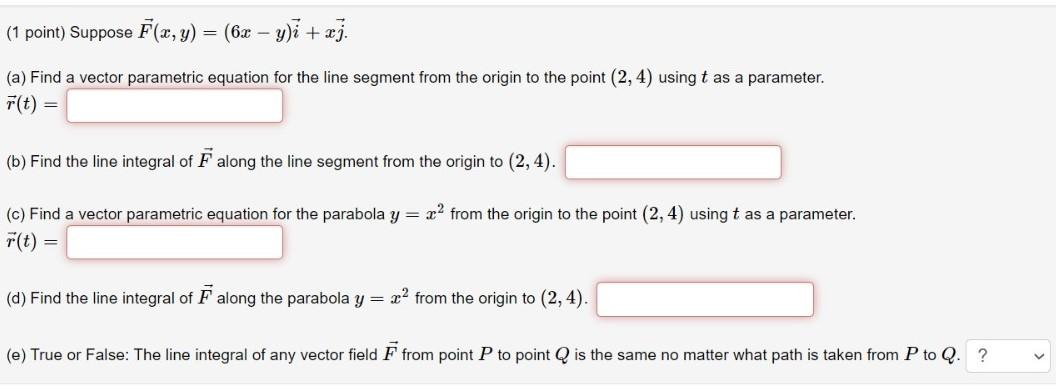### Create an Account

Already have account?

### Forgot Your Password ?

Home / Questions / (1 point) Suppose F(x, y) = (6x - y)i + xj. (a) Find a vector parametric equation for the ...

# (1 point) Suppose F(x, y) = (6x - y)i + xj. (a) Find a vector parametric equation for the line segment from the origin to the point (2, 4) using t as a parameter. F(t) = (b) Find the line integral of

(1 point) Suppose F(x, y) = (6x - y)i + xj. (a) Find a vector parametric equation for the line segment from the origin to the point (2, 4) using t as a parameter. F(t) = (b) Find the line integral of F along the line segment from the origin to (2, 4). (c) Find a vector parametric equation for the parabola y = x2 from the origin to the point (2,4) using t as a parameter. r(t) = (d) Find the line integral of F along the parabola y = x2 from the origin to (2,4). (e) True or False: The line integral of any vector field F from point P to point Q is the same no matter what path is taken from P to Q. ? VApr 14 2021 View more View Less

#### Answer (Solved)Subscribe To Get Solution